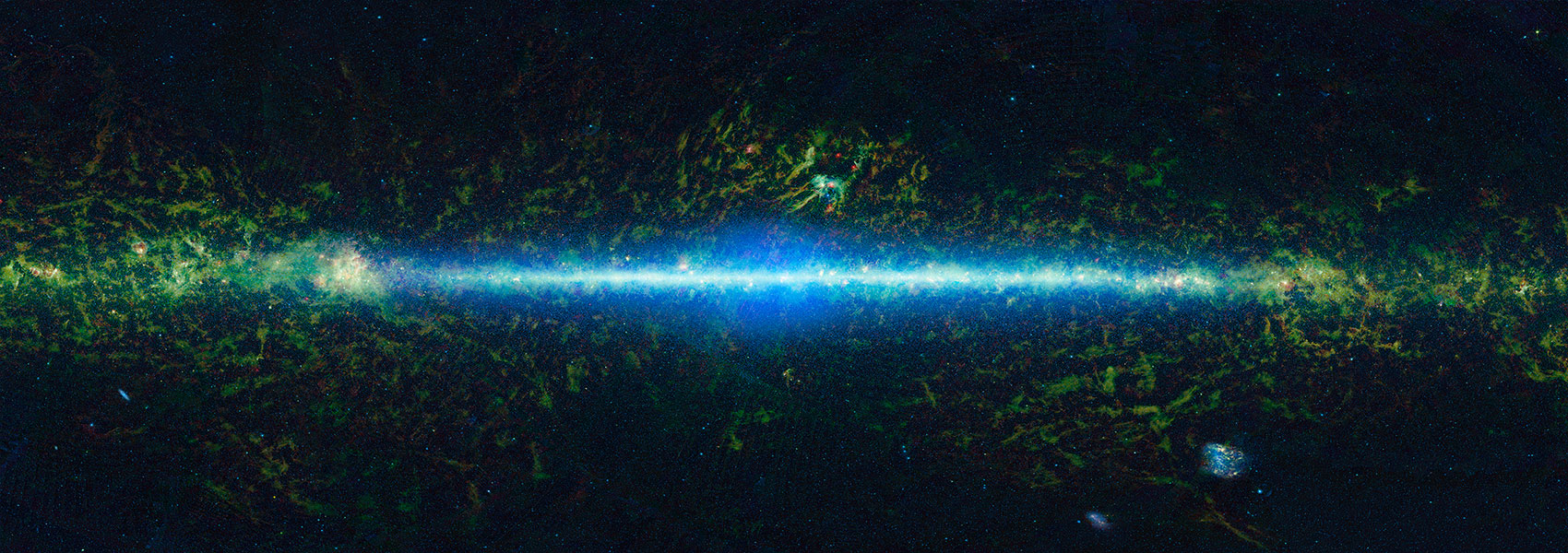# Exploring uncertainties in dark energy constraints using current observational data with Planck 2015 distance priors

October 2016 • 2016PhRvD..94h3521W

Authors • Wang, Yun • Dai, Mi

Abstract • We present the distance priors that we have derived from the 2015 Planck data, and use these in combination with the latest observational data from Type Ia supernovae (SNe Ia) and galaxy clustering, to explore the systematic uncertainties in dark energy constraints. We use the joint light-curve analysis (JLA) set of 740 SNe Ia, galaxy clustering measurements of H (z )s and DA(z )/s (where s is the sound horizon at the drag epoch) from the Sloan Digital Sky Survey (SDSS) at z =0.32 and z =0.57 (BOSS DR12). We find that the combined dark energy constraints are insensitive to the assumptions made in the galaxy clustering measurements (whether they are for BAO only or marginalized over RSD), which indicates that as the analysis of galaxy clustering data becomes more accurate and robust, the systematic uncertainties are reduced. On the other hand, we find that flux-averaging SNe Ia at z ≥0.5 significantly tightens the dark energy constraints and excludes a flat universe with a cosmological constant at 68% confidence level, assuming a dark energy equation of state linear in the cosmic scale factor. Flux averaging has the most significant effect when we allow the dark energy density function X (z ) to be a free function given by the cubic spline of its values at z =0 , 1/3 , 2/3 , 1; the measured X (z ) deviates from a cosmological constant at more than 95% confidence level for 0.4 ≲z ≲0.7 . Since flux averaging reduces the bias in the SN distance measurements, this may be an indication that we have arrived in the era when the SN distance measurements are limited by systematic uncertainties.